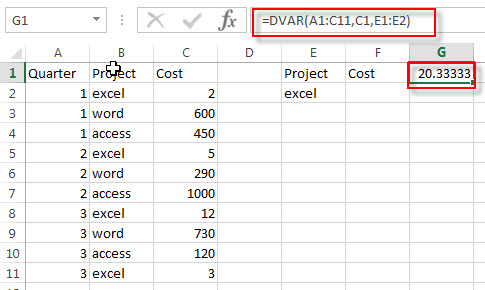## Excel DVAR Function

This post will guide you how to use Excel DVAR function with syntax and examples in Microsoft excel.

### Description

The Excel DVAR Function will get the variance of a population based on a sample of numbers in a column in a list or database based on a given criteria.

The DVAR function is a build-in function in Microsoft Excel and it is categorized as a Database Function.

The DVAR function is available in Excel 2016, Excel 2013, Excel 2010, Excel 2007, Excel 2003, Excel XP, Excel 2000, Excel 2011 for Mac.

### Syntax

The syntax of the DVAR function is as below:

`= DVAR (database, field, criteria)`

Where the DVAR function arguments are:

• Database -This is a required argument. The range of cells that containing the database.
• Field – This is a required argument. The column with database that you want the minimum of.
• Criteria – The range of cells that contains the conditions that you specify.

### Excel DVAR Function Examples

The below examples will show you how to use Excel DVAR Function to get the variance of a population based on a sample by using the numbers in a column in a list or database that match a given criteria.

#1 to get the sample variance of cost for the numbers in a range A1:C11,  using the following formula:

`=DVAR(A1:C11,C1,E1:E2)`### Related Functions

• Excel DVARP Function
The Excel DVARP Function will get the variance of a population based on the entire population of numbers in a column in a list or database based on a given criteria. The syntax of the DVARP function is as below:= DVARP(database, field, criteria)…